# Need help with NPV formulaHi Everyone,

I was applying discount percentage of 4% to a cash value of -193 , I feel that my value is incorrect please see attached screenshot.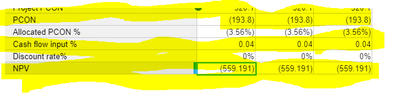My formula is NPV(Cash flow input %, PCON). Could you please help me what I am doing wrong here.

Regards,

Manisha

Tagged:

•I was expecting NPV to be -185.65

•Hey - Can you add a line item which is boolean formatted and then check the cash flow period from which npv calculation has to happen and then make it part of formula and see if that corrects.

•Is your PCON always a negative value for each period (cash out?). NPV function needs at least one negative value (cash out) and at least one positive value (cash in).

Your NPV will correct itself once you enter the correct cash flows by period.

I think by reading your use case you're expecting .04/12 cash in each month or .646 after your initial investment of -193.8. To get that result you'll need to set up your cash flows like this. We get a number much closer to what you were expecting.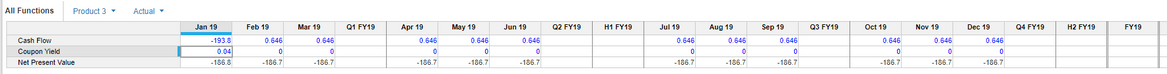If you have a more complex time series, you can use the 3rd and 4th variable in the function. You can read about it in this article.

https://help.anaplan.com/anapedia/Content/Calculation_Functions/All/NPV.html

•Hi Jared,

Thank you for the response! The time scale i use is year, should i convert it to monthly values and assign the numbers in such way?

Thanks,

Manisha

•Before we go there, can you describe the use case?

• What is the initial investment? 193.8?
• What is the annual return? 4%?
• What is the cash flow frequency? Monthly? Yearly?

From there, you can set up your formula.

The NPV function does not need a time dimension. You would only use the time dimension as a convenience to you so you can plan your cash flows.

Here's an Annual Example:

I invest 100 at 4% annual return and at the end of 3 years I get 112.5.

The NPV is, as expected, 4.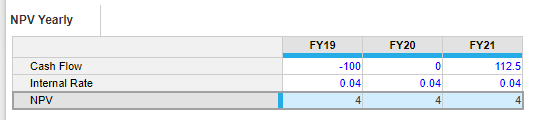'

Try breaking up your use case like this: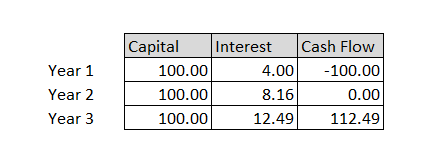•I have different PCON's for each of the 3 years and I am applying 4% cashflow% for all 3 years.

My NPV is same as my PCON, could you let me know what am I doing wrong here.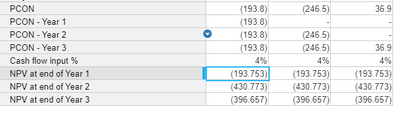Regards,

Manisha

•In order to get the exact value, you have to use "POWER(1+ Cashflow rate %,N)"  formula in order to match Excel's NPV formula, where N is the no of year.

I have tried to replicate your problem below:

I have used the above logic to get the correct NPV.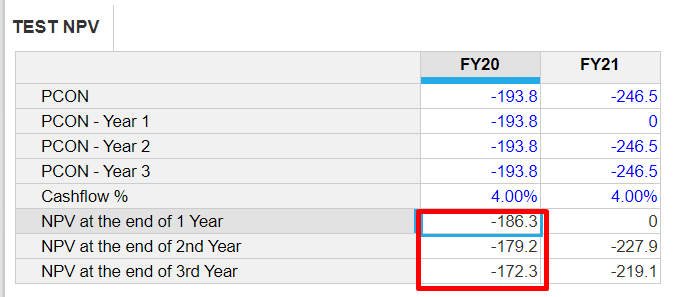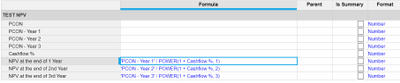Excel calculation: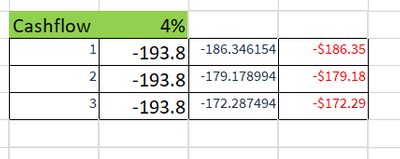Hope this helps!

Thanks

Akhtar

•Hi Akhtar,

I believe you're right! Will try and let you know. Thanks a ton!